# Similarity of two triangles

The KLM triangle has a side length of k = 6.3cm, l = 8.1cm, m = 11.1cm. The triangle XYZ has a side length of x = 8.4cm, y = 10.8cm, z = 14.8cm. Are triangle KLM and XYZ similar? (write 0 if not, if yes, find and write the coefficient of a similarity)

q =  1.3333

### Step-by-step explanation: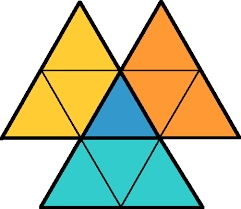Did you find an error or inaccuracy? Feel free to write us. Thank you!Tips to related online calculators
Do you want to convert length units?

#### You need to know the following knowledge to solve this word math problem:

We encourage you to watch this tutorial video on this math problem:

## Related math problems and questions:

• Similarity coefficientIn the triangle TMA the length of the sides is t = 5cm, m = 3.5cm, a = 6.2cm. Another similar triangle has side lengths of 6.65 cm, 11.78 cm, 9.5 cm. Determine the similarity coefficient of these triangles and assign similar sides to each other.
• Similar trianglesThe triangles ABC and XYZ are similar. Find the missing lengths of the sides of the triangles. a) a = 5 cm b = 8 cm x = 7.5 cm z = 9 cm b) a = 9 cm c = 12 cm y = 10 cm z = 8 cm c) b = 4 cm c = 8 cm x = 4.5 cm z = 6 cm
• SimilarityRectangle ABCD has dimensions of 7 cm and 6 cm. Rectangle PQRS has dimensions 14 cm and 12 cm. Determine coefficient of the similarity k of the rectangles, if they aren't similar enter zero as the coefficient of similarity.
• SimilarityABC is a triangle wherein a = 4 cm, b = 6 cm, c = 8 cm. Is it similar to the triangle DEF: d = 3 cm, e = 4.5 cm, f = 6 cm? If so, determine the ratio of similarity.
• Similarity coefficientThe ratio of similarity of two equilateral triangles is 3.5 (ie 7:2). The length of the side of smaller triangle is 2.4 cm. Calculate the perimeter and area of ​​the larger triangle.
• The triangles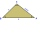The triangles KLM and ABC are given, which are similar to each other. Calculate the lengths of the remaining sides of the triangle KLM, if the lengths of the sides are a = 7 b = 5.6 c = 4.9 k = 5
• What is 10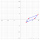What is this The area of a parallelogram that has vertices with the coordinates (0, 0), (4, 0),(5, 3), and (1, 3)?
• Sides od triangleSides of the triangle ABC has length 4 cm, 5 cm and 7 cm. Construct triangle A'B'C' that are similar to triangle ABC which has a circumference of 12 cm.
• Two similar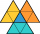Two similar triangles, one has a circumference of 100 cm, the second has sides successively 8 cm, 14 cm, 18 cm longer than the first. Find the lengths of its sides.
• Similarity coefficient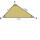The triangles ABC and A "B" C "are similar to the similarity coefficient 2. The sizes of the angles of the triangle ABC are α = 35° and β = 48°. Find the magnitudes of all angles of triangle A "B" C ".Show that the quadrilateral with vertices P1(0,1), P2(4,2) P3(3,6) P4(-5,4) has two right triangles.Subtract the following decimals. a. 0.98 - 0.053 b. 0.67 - 0.4 c. 0.3 - 0.002 d. 3.2 - .789 e. 6.53 - 4.298 f. 6 - 4.32 g. 7 - 3.574 h. 4.83 - 1.8 i. 3.7 - 1.8 j. 16.17 - 11.632How many right triangles we can construct from line segments 3,4,5,6,8,10,12,13,15,17 cm long? (Do not forget to the triangle inequality).The pit has the shape of a truncated pyramid with a rectangular base and is 0.8 m deep. The pit's length and width are the top 3 × 1.5 m bottom 1 m × 0.5 m. To paint one square meter of the pit we use 0.6 l of green color. How many liters of paint are neeHow many 7-digit telephone numbers can be compiled from the digits 0,1,2,..,8,9 that no digit is repeated?In the triangle DEF is DE = 21cm, EF = 14.7cm, DF = 28cm. The triangle D´E´F´ is similar to the triangle DEF. Calculate the lengths of the sides of the triangle D´E´F´ if the similarity coefficient is one-seventh.Which group of decimals is in order from least to greatest? A) 1.04, 0.39, 0.8, 2.1, 0.09 B) 2.1, 1.04, 0.39, 0.8, 0.09 C) 0.09, 0.39, 0.8, 1.04, 2.1 D) 0.09, 0.39, 0.8, 2.1, 1.04